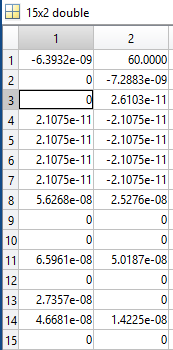# CVX program solved without solving constraint

``````p = 0.5;
assets = 2;
cases = 2;
timePeriod = 4;
childPerNode = 2;
nodes = power(2, timePeriod) - 1;
initialWealth = 60;
targetWealth = 80;
prob = power(p, timePeriod - 1);
randomReturn = zeros(cases, assets);
randomReturn(1, 1) = 1.25;
randomReturn(1, 2) = 1.14;
randomReturn(2, 1) = 1.06;
randomReturn(2, 2) = 1.12;
g = 1.1;
r = 1.3;
cvx_begin
variable x(nodes, assets)
variable y(nodes)
variable wplus(nodes)
variable wminus(nodes)
z = 0;
for i = power(2, timePeriod - 1) : power(2, timePeriod) - 1
z = z + prob * (g * wplus(i) - r * wminus(i));
end
maximize(z)
subject to
y == sum(x, 2);
y(1) == initialWealth;
for i=1:floor(nodes/4)
%if 2 * i <= floor(nodes/2)
randomReturn(1, :) * transpose(x(i)) - y(2 * i) == 0;
randomReturn(2, :) * transpose(x(i)) == y(2 * i + 1);
%end
end
for i = power(2, timePeriod - 2): power(2, timePeriod - 1) - 1
randomReturn(1, :) * transpose(x(i)) == targetWealth + wplus(2*i) - wminus(2*i);
randomReturn(2, :) * transpose(x(i)) == targetWealth + wplus(2*i + 1) - wminus(2*i + 1);
end
x >= 0;
wplus >= 0;
wminus >= 0;
cvx_end
``````

Hello,

I am trying to implement algorithm given in page 5 of the link: http://scjournal.ius.edu.ba/index.php/scjournal/article/viewFile/60/60.

Following is my variable x.I have a constraint and lots of similar constraints which are not satisfied:
1.25 * x(1, 1) + 1.14 * x(1, 2) = x(2,1) + x(2,2).

I don’t know why these constraints are not satisfied.
Only the constraint x(1,1) + x(1,2) = 60 is working. Need some help!!

I didn’t check whether your model makes sense. But I did run it with multiple solvers, and the optimal solution satisfied constraints within tolerance. You have no guarantee the constraints will be exactly satisfied. For instance x(1,1) = -6.3912e-09 is within allowable tolerance of being nonnegative. In fact, the exact optimal `x(1,:) = zeros(15,1)` corresponding to your “solution”. However, your model is rather degenerate and has multiple optimal solutions all achieving the optimal objective value of -104.

If you have a solution which has a constraint violation of more than 1e-8, please show us the output and the amount of the constraint violation.

Hi Mark,

Thanks for the reply. x(1, 1) = -6.3912e-09 is not a problem. But, the issue is it is not satisfying the following constraints (extracted from the full code above):

y == sum(x, 2);
y(1) == initialWealth;
for i=1:floor(nodes/4)
%if 2 * i <= floor(nodes/2)
randomReturn(1, : ) * transpose(x(i)) - y(2 * i) == 0;
randomReturn(2, : ) * transpose(x(i)) == y(2 * i + 1);
%end
end

The first line says y(i) = x(i, 1) + x(i, 2) for all i = 1, 2, …, 15.
Now, y(1) = x(1, 1) + x(1, 2) = 60 (initial wealth). This constraint is satisfied.
In the for loop when i = 1, the constraint becomes as follows after putting the value of variables:
1.25 * x(1, 1) + 1.14 * x(1, 2) = y(2) = x(2, 1) + x(2, 2). But, this constraint is not satisfied since 1.14 * 60 != -7.28 * 10 ^(-9).